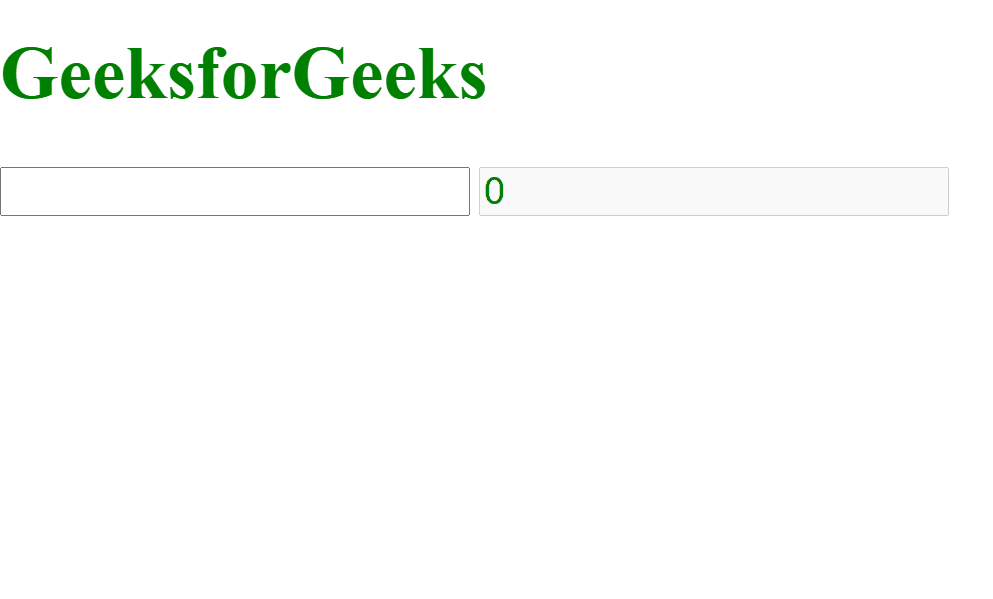GeeksforGeeks App
Open AppBrowser
Continue

# How to count words in real time using jQuery ?

In this article, we will learn how to count words in real-time using jQuery. We have an input field, and when we type, we get the number of words in the input in real time.

Approach: First, we select the input field and store its value in a variable. We call a function called wordCount() when a keyboard key is released that counts the number of words in the input field. To count the number of words in the input field, we split the value by space using the jQuery split() method and then count the number of words.

Code snippet: The following is the implementation of the method wordCount().

```function wordCount( field ) {
let number = 0;

// Split the value of input by
// space to count the words
let matches = \$(field).val().split(" ");

// Count number of words
number = matches.filter(function (word) {
return word.length > 0;
}).length;

// final number of words
\$("#final").val(number);
}```

Example: Below is the full implementation of the above approach.

HTML code:

## HTML

 ```<``html` `lang``=``"en"``>` `<``head``>``    ``<``script` `src``=``"https://code.jquery.com/jquery-3.5.0.js"``>``    ````    ``<``style``>``        ``body {``            ``color: green;``            ``font-size: 30px;``        ``}` `        ``input {``            ``font-size: 30px;``            ``color: green;``        ``}``    ````` `<``body``>``    ``<``h1``>GeeksforGeeks``    ``<``input` `type``=``"text"` `id``=``"inp"` `/>``    ``<``input` `type``=``"text"``           ``id``=``"final"` `disabled />``    ``<``script``>``        ``function wordCount(field) {``            ``let number = 0;``            ``// Split the value of input by``            ``// space to count the words``            ``let matches = \$(field).val().split(" ");``            ``// Count number of words``            ``number = matches.filter(function (word) {``                ``return word.length > 0;``            ``}).length;``            ``// Final number of words``            ``\$("#final").val(number);``        ``}``        ``\$(function () {``            ``\$("input[type='text']:not(:disabled)")``                ``.each(function () {``                    ``let input = "#" + this.id;``                    ``// Count words when keyboard``                    ``// key is released``                    ``\$(this).keyup(function () {``                        ``wordCount(input);``                    ``});``                ``});``        ``});``    ````` ``

Output:How to count words in real time using jQuery ?

My Personal Notes arrow_drop_up
Related Tutorials